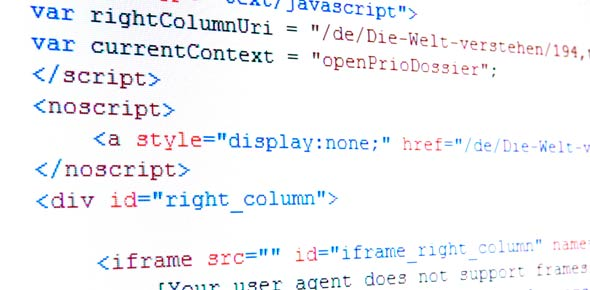# Programming Quiz - 3rd Form Students Sjc

20 Questions | Attempts: 110
ShareSettingsTests the students knowledge on programming algorithms and concepts. Created by Miss Stacy Sutherland for my third form students.

• 1.
Which of the following is an assignment statement?
• A.

Sum

• B.

Print

• C.

• D.

Write

• 2.
The process of searching, identifying and correcting errors in a program is called:
• A.

Correcting

• B.

Debugging

• C.

Dry run

• D.

Testing

• 3.
Which of the following logical (relational) operators are used to represent not equal to in an algorithm?
• A.

• B.

• C.

• D.

• 4.
Instructions that can be repeated are called:
• A.

FOR

• B.

WHILE

• C.

LOOP

• D.

VARIABLE

• 5.
Every _______ statement must begin with a variable, followed by the equal sign and then a variable, constant or a calculation.
• A.

INPUT

• B.

ASSIGNMENT

• C.

OUTPUT

• D.

SELECTION

• 6.
A manual traversal of an algorithm, is often referred to as:
• A.

DRY RUN

• B.

LOGICAL TESTING

• C.

PSEUDOCODE

• D.

TRACE TABLE

• 7.
An example of a low level language is :
• A.

Assembly level language

• B.

4GLS

• C.

Natural Language

• D.

Procedural language

• 8.
What is the data type that uses the % character at the end of its variable name in a structured algorithm?
• A.

Currency

• B.

Float

• C.

Integer

• D.

String

• 9.
What is referred to as an identifier that stores a changeable value in a particular location in memory?
• A.

Constant

• B.

String

• C.

Variable

• D.

Array

• 10.
Which of the following is an example of a conditional loop?
• A.

For – Next

• B.

If-then-else

• C.

While-end while

• D.

For-end for

• 11.
Within a conditional loop algorithm, the termination constant is often referred to as:
• A.

Loop variable

• B.

Conditional variable

• C.

Dummy value

• D.

Loop constant

• 12.
Algorithms can be written in different forms except
• A.

Pseudocode

• B.

Flowchart

• C.

Defining Diagram

• D.

None of the Above

• 13.
Data in an algorithm that remains the same is considered to be :
• A.

Constant

• B.

Variable

• C.

Integer

• D.

String

• 14.
All algorithms have a defined structure. Which of the following state the parts of an algorithm in proper order?
• A.

Sequence, Repetition, Selection

• B.

Loop, Do, While, End

• C.

• D.

Simple, Conditional, Loop.

• 15.
Which of the following is the proper code to be written to read and accept a variable NAME?
• A.

Print “Enter a name:” Input name%

• B.

Print “Enter name: Input name\$

• C.

Print “Enter a name:” Input Name\$

• D.

Print Enter a name: Input “Name\$”

• 16.
Mistakes that are made by a non-fulfillment to the grammatical rules of the programming language are called:
• A.

Debugging

• B.

Logic errors

• C.

Run-time errors

• D.

Syntax errors

• 17.
If a program survives various unexpected events, such as incorrect or invalid data input it is said to be
• A.

Pseudocode

• B.

Robust

• C.

Executed

• D.

Compiled

• 18.
A manual traversal of an algorithm is called:
• A.

Trace table

• B.

Dry Run

• C.

Dummy Value

• D.

Condtional Loop

• 19.
Instructions that are repeated a predetermined or set amount of times is often referred to as:
• A.

Condtional Loop

• B.

While Loop

• C.

Loop

• D.

Counted Loop

• 20.
An example of variable initialization is:
• A.

Total =total+12

• B.

Count=0

• C.

Sum=num1+num2

• D.

Temp = 1 to 7

## Related TopicsBack to top
×

Wait!
Here's an interesting quiz for you.